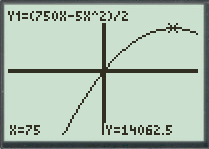# The function that models the total area of the four pens and largest possible total area of the four pens.### Precalculus: Mathematics for Calcu...

6th Edition
Stewart + 5 others
Publisher: Cengage Learning
ISBN: 9780840068071### Precalculus: Mathematics for Calcu...

6th Edition
Stewart + 5 others
Publisher: Cengage Learning
ISBN: 9780840068071

#### Solutions

Chapter 3.1, Problem 72E
To determine

## To find: The function that models the total area of the four pens and largest possible total area of the four pens.

Expert Solution

The function that models the total area of the four pens is equal to g(x)=750x5x22 and largest possible total area of the four pens is equal to 14062.5ft2 .

### Explanation of Solution

Given information:

A rancher with 750ft of fencing wants to enclose a rectangular area and then divide it into four pens with fencing parallel to one side of rectangle(see figure).Calculation:

Assume that the breadth of the rectangular region is equal to xft and as the sides along the breadth are 5 . So, the total fence for the five sides along breadth is equal to 5xft .

The fence left is used to fence 2 sides along the length of rectangular region. The left fence is equal to 7505xft . So, the length of the rectangular region is equal to 7505x2ft .

Use the formula for the area of the rectangular region.

A=L×BA=(7505x2)×xA=750x5x22

So, the area function of the rectangular region is given by g(x)=750x5x22 . To maximize the area of the rectangular region graph the area function g(x)=750x5x22 .

To graph a function y=750x5x22 , follow the steps using graphing calculator.

First press “ON” button on graphical calculator, press Y= key and enter right hand side of the equation y=750x5x22 after the symbol Y1 . Enter the keystrokes (750X5X^2)÷2 .

The display will show the equation,

Y1=(750X5X^2)/2

Now, press the GRAPH key and TRACE key to produce the graph of given function in standard window as shown in Figure (1).Figure (1)

As observed from the graph, the area function has maximum value equal to 14062.5ft2 at x=75 .

Therefore, the function that models the total area of the four pens is equal to g(x)=750x5x22 and largest possible total area of the four pens is equal to 14062.5ft2 .

### Have a homework question?

Subscribe to bartleby learn! Ask subject matter experts 30 homework questions each month. Plus, you’ll have access to millions of step-by-step textbook answers!